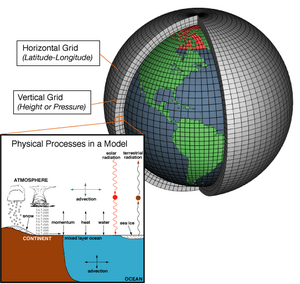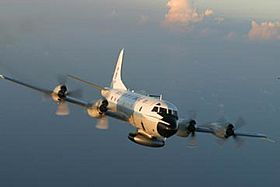# Numerical weather prediction facts for kids

Kids Encyclopedia FactsWeather models use systems of differential equations based on the laws of physics, fluid motion, and chemistry, and use a coordinate system which divides the planet into a 3D grid. Winds, heat transfer, solar radiation, relative humidity, and surface hydrology are calculated within each grid cell, and the interactions with neighboring cells are used to calculate atmospheric properties in the future.Weather reconnaissance aircraft, such as this WP-3D Orion, provide data that is then used in numerical weather forecasts.

Numerical weather prediction is the way weather forecasts are made. This is done using computer models of the atmosphere. Such models describe the current weather conditions, and how they change over time using equations. Using the current weather conditions, the equations can be solved, or approximated to tell what the weather will be like in the near future. The relevant physical parameters, such as pressure, temperature, the direction and the speed of the wind are taken to be functions of time. These are modelled with a system of partial differential equations. This is a dynamic system that is solved numerically. Most of these equations are implemented using FORTRAN. The equations are approximated. Since this takes a lot of computing power or time, supercomputers are used for this task, most of the time.

## Basic idea

The atmosphere is modelled as a fluid. The basic idea of numerical weather prediction is to sample the state of the fluid at a given time. The equations of fluid dynamics and thermodynamics can then be used to estimate the state of the fluid at some time in the future.

## Local weather prediction

The results are usually too inaccurate to be used for predicting the weather at any place. For this reason, meteorologists check the values, and compare them to historical data. In other words, they use the data to help produce the weather forecast.

Model Output Statistics is a statistical model that was developed in the 1960s and 1970s. It uses regression analysis for a fully automated forecast. With it, historical data is analysed automatically. One of its applications is called Direct Model Output. MOS uses both historical data and statistical modeling. Predictions beyond about six hours are unreliable.

Another well-known model is called Global Forecast System (GFS), which is run by the US weather service, NOAA. It gives a forecast four times a day. Since the information is free, the GFS is often used, especially by smaller weather stations.

## Ensembles

The atmosphere is a chaotic system. A small change of the input values does not necessarily lead to a small change of the output. This is due to the equations of fluid dynamics that are involved. These equations involved are solved or approximated once with the parameters that were observed. This is done several times more, with parameters that are based on the observed values, but which have been changed slightly. Because the computing power is limited, the "resolution" of such a model is more coarse. Once all the calculations are finished, they are compared to one another. Calculated results that are "similar" indicate that the prognosis is relatively good. In some cases, this means that it is possible to accurately predict the weather for a time of about ten days; in other cases a prediction over even a few days may be difficult.

## Images for kidsNumerical weather prediction Facts for Kids. Kiddle Encyclopedia.